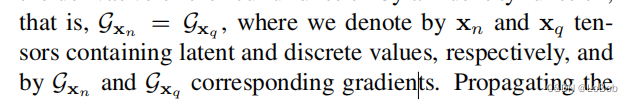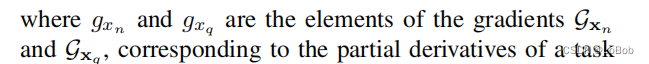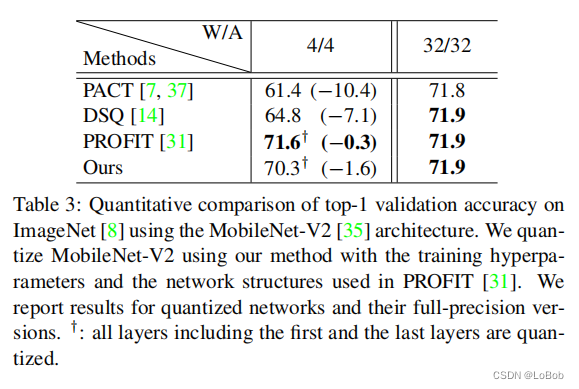# EWSG 基于元素级梯度缩放_LoBob

EWGS：基于(element-wise)元素级梯度缩放的网络量化

## 1、EWGS公式¶

1、考虑了quantize输入和输出的误差，自适应的放大或者缩小梯度

2、将比例因子与任务损失二阶导数联系起来，使用Hessian矩阵的迹来估计因子

3、不需要广泛的超参数搜索。

lu是一组fp32数的最大值和最小值，下面的公式就是把输入x映射到0，1的浮点数值

x_{n}=\operatorname{clip}\left(\frac{x-l}{u-l},0,1\right)(1)

x_{q}=\frac{\operatorname{round}\left(\left(2^{b}-1\right)x_{n}\right)}{2^{b}-1}(2)

Q_{W}(x)=2\left(x_{q}-0.5\right),Q_{A}(x)=x_{q}(3)

g_{x_{n}}=g_{x_{q}}\left(1+\delta\operatorname{sign}\left(g_{x_{q}}\right)\left(x_{n}-x_{q}\right)\right)(4)

STE是这样的，$\mathcal{G}_{\mathbf{x}_{n}}=\mathcal{G}_{\mathbf{x}_{q}}$, 直接将导数为0或者不可导的变成了1，直接直通。 因为这些下标太难打了，我直接截图了。一个是公式(1)的梯度，一个是公式(2)的梯度。δ是大于0的数值，当δ等于0的时候，EWSG就是STE了。STE是次优的原因：

（1）多个$x_n$可以产生相同的$x_q$，大白话就是多个fp32的数值变成同一个int值；

（2）$x_n$提供的相同梯度对每个$x_q$都有不同的影响，大白话就是虽然梯度$x_n$的一样的，但是对应的$x_q$是不一样的，这个就是mismatch的问题。

## 2、如何确定δ数值，基于海森矩阵的方法¶

\begin{aligned} g_{x_{n}}&=g_{x_{q}}+\frac{g_{x_{n}}-g_{x_{q}}}{x_{n}-x_{q}}\left(x_{n}-x_{q}\right)\\ &=g_{x_{q}}+\frac{g_{x_{q}+\epsilon}-g_{x_{q}}}{\epsilon}\left(x_{n}-x_{q}\right)\\ \end{aligned}\\
g_{x_{n}}\approx g_{x_{q}}+g_{x_{q}}^{\prime}\left(x_{n}-x_{q}\right)
g_{x_{n}}\approx g_{x_{q}}\left(1+\frac{g_{x_{q}}^{\prime}}{\left|g_{x_{q}}\right|}\operatorname{sign}\left(g_{x_{q}}\right)\left(x_{n}-x_{q}\right)\right) g_{x_{q}}^{\prime}=\frac{\partial g_{x_{q}}}{\partial x_{q}}

g_{x_{q}}^{\prime}=\frac{\partial g_{x_{q}}}{\partial x_{q}}

\delta=\frac{g_{x_{q}}^{\prime}}{\left|g_{x_{q}}\right|}

\mathbb{E}\left[\mathbf{v} \mathbf{v}^{T}\right]=I

\begin{aligned} \operatorname{Tr}(H) &=\operatorname{Tr}(H I)=\operatorname{Tr}\left(H \mathbb{E}\left[\mathbf{v}^{T}\right]\right) \\ &=\mathbb{E}\left[\operatorname{Tr}\left(H \mathbf{v} \mathbf{v}^{T}\right)\right]=\mathbb{E}\left[\mathbf{v}^{T} H \mathbf{v}\right] \end{aligned}

\delta=\frac{\operatorname{Tr}(H) / N}{G} (5)

3 \sigma\left(\mathcal{G}_{\mathbf{x}_{q}}\right)

## 3、实验¶## 4、总结¶

EWGS结果没有超过前人的工作，我比较欣赏的是他的方法很简单，而且不给推理增加负担，是篇好工作。CVPR2020也有一篇做量化训练的时候修改梯度的，UnifiedINT8，通过修改梯度的方向和数值来缓解mismatch带来的影响。但EWGS从数学上个人觉得更加可解释和合理。故记录一下。